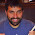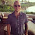## Tuesday, March 01, 2011

### Clojure: if-let and when-let

I'm a fan of if-let and when-let. Both can be helpful in creating succinct, readable Clojure code. From the documentation:
If test is true, evaluates then with binding-form bound to the value of test, if not, yields else
A quick example should demonstrate the behavior.
`user=> (if-let [a :anything] a "no")    :anythinguser=> (if-let [a nil] a "no")      "no"user=> (if-let [a false] a "no")"no"`
The above example demonstrates a simple case where a truthy value causes the "then" form to be returned, and a falsey value (nil or false) causes the "else" form to be returned. The example also shows that "a" is bound to the value :anything and can be used within the "then".

The following example shows that you can put code in the "then" and "else" statement's as well.
`user=> (if-let [a 4] (+ a 4) (+ 10 10)) 8user=> (if-let [a nil] (+ a 4) (+ 10 10))20`
Just like above, when "a" is truthy the value is bound and it can be used as desired in the "then".

The when-let function behaves basically the same way, except there is no "else".
`user=> (when-let [a 9] (+ a 4))  13user=> (when-let [a nil] (+ a 4))niluser=> (when-let [a 9] (println a) (+ a 4))  913`
The example demonstrates that then "then" case is very similar to if-let; however, if the test fails the when-let function simply returns nil. The last when-let example also shows how it slightly differs from an if-let: you can pass as many forms to the "then" as you'd like. If the test evaluates to true, all of the additional forms will be evaluated.

There are a few things worth knowing about if-let and when-let with respect to the bindings. Both if-let and when-let require a vector for their bindings, and there must be exactly two forms in the binding vector. The following example shows Clojure's response if you choose not to follow those rules.
`user=> (when-let (a 9) (println a))java.lang.IllegalArgumentException: when-let requires a vector for its binding (NO_SOURCE_FILE:0)user=> (when-let nil (println "hi"))java.lang.IllegalArgumentException: when-let requires a vector for its binding (NO_SOURCE_FILE:0)user=> (when-let [a 9 b nil] (println a b))java.lang.IllegalArgumentException: when-let requires exactly 2 forms in binding vector (NO_SOURCE_FILE:0)`
It's nice to know if-let and when-let, but they aren't exactly hard concepts to follow. Once someone points them out to you, I expect you'll be able to easily use them in your code without much effort.

However, what is the impact of destructuring on if-let and when-let? I know what I expected, but I thought it was worth a quick trip to the REPL to make sure my assumptions were correct. The following code shows that as long as the value being bound is truthy the "then" will be executed - destructuring values have their normal behavior and do no effect execution flow.
`user=> (when-let [{a :a} {:a 1}] [a])user=> (when-let [{a :a} {}] [a])    [nil]user=> (when-let [{a :a} {:a false}] [a])[false]user=> (when-let [{a :a} nil] [a])       nil`
As you would expect the when-let is consistent and the destructuring behavior is also consistent.

if-let and when-let are good, give them a shot.

1.wI actually do not understand how these two:

user=> (when-let [{a :a} {}] [a])
[nil]
user=> (when-let [{a :a} {:a false}] [a])
[false]

I would think both of those would return nil. I don't understand how those destructuring binds result in truthy values.

I see that this is indeed the behavior though. Do you have any further insight on this? I didn't think it was intuitive.

2.When you do a when-let you're code is conditional on what expression you have on the right. If you also choose to destructure that value the destructured locals will have no impact on the conditional.

In both cases you're expecting when-let to decide based on the destructured value; however it's deciding based on the undestructured value {} in the first example and {:a false} in the second example.

Note: Only a member of this blog may post a comment.